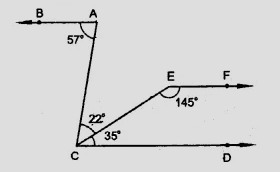# In the figure, show that $AB \parallel EF$."

To do:

We have to show that $AB \parallel EF$.

Solution:

From the figure,

$\angle BAC = 57^o, \angle ACE = 22^o$

$\angle ECD = 35^o$ and $\angle CEF =145^o$

$\angle ECD + \angle CEF = 35^o + 145^o$

$= 180^o$

$\angle ECD$ and $\angle CEF$ are co-interior angles.

Therefore,

$EF \parallel CD$

$\angle BAC = 57^o$

$\angle ACD = \angle ACE + \angle ECD$

$= 22^o+35^o$

$= 57^o$

This implies,

$AB \parallel CD$

$EF \parallel CD$ and $AB \parallel CD$

Therefore,

$AB \parallel EF$.

Hence proved.